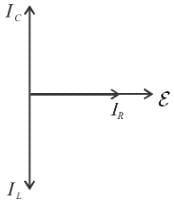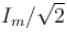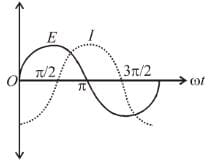Alternating Current MCQ Level - 1

# Alternating Current MCQ Level - 1

Test Description

## 10 Questions MCQ Test Topic wise Tests for IIT JAM Physics | Alternating Current MCQ Level - 1

Alternating Current MCQ Level - 1 for Physics 2023 is part of Topic wise Tests for IIT JAM Physics preparation. The Alternating Current MCQ Level - 1 questions and answers have been prepared according to the Physics exam syllabus.The Alternating Current MCQ Level - 1 MCQs are made for Physics 2023 Exam. Find important definitions, questions, notes, meanings, examples, exercises, MCQs and online tests for Alternating Current MCQ Level - 1 below.
Solutions of Alternating Current MCQ Level - 1 questions in English are available as part of our Topic wise Tests for IIT JAM Physics for Physics & Alternating Current MCQ Level - 1 solutions in Hindi for Topic wise Tests for IIT JAM Physics course. Download more important topics, notes, lectures and mock test series for Physics Exam by signing up for free. Attempt Alternating Current MCQ Level - 1 | 10 questions in 30 minutes | Mock test for Physics preparation | Free important questions MCQ to study Topic wise Tests for IIT JAM Physics for Physics Exam | Download free PDF with solutions
 1 Crore+ students have signed up on EduRev. Have you?
Alternating Current MCQ Level - 1 - Question 1

### Which of the following has different dimension from the other three,

Detailed Solution for Alternating Current MCQ Level - 1 - Question 1

Alternating Current MCQ Level - 1 - Question 2

### An alternating emf is applied across a parallel combination of a resistance R, capacitance C and an induction L. If IR, IL, IC are the currents through R, L and C respectively, then the diagram which correctly represents the phase relationship among IR, IL, IC and source emf ε is the given by

Detailed Solution for Alternating Current MCQ Level - 1 - Question 2

IL lags behind IR by a phase of π/2
IC lags behind IR by a phase of π/2Alternating Current MCQ Level - 1 - Question 3

### The average power dissipated is pure inductance is

Alternating Current MCQ Level - 1 - Question 4

The value of current at half power points is

Detailed Solution for Alternating Current MCQ Level - 1 - Question 4

The correct answer is:Alternating Current MCQ Level - 1 - Question 5

A DC ammeter cannot measure alternating current value

Alternating Current MCQ Level - 1 - Question 6

The time required for a 50Hz alternating current to increase from zero to 70.7% of its peak value is

Detailed Solution for Alternating Current MCQ Level - 1 - Question 6

Solution :- 1/(50×8)

= 1/400sec

= 2.5ms

Alternating Current MCQ Level - 1 - Question 7

The variation of the instantaneous current I and the instantaneous emf E in a circuit is as shown in figure. Which of the following statements is correctDetailed Solution for Alternating Current MCQ Level - 1 - Question 7

Alternating Current MCQ Level - 1 - Question 8

The term cos φ in an AC circuit is called

Detailed Solution for Alternating Current MCQ Level - 1 - Question 8

The correct answer is: power factor

Alternating Current MCQ Level - 1 - Question 9

In a series LCR circuit containing an AC voltage source of frequency ω, current and voltage V. Then for the given resistance, the correct statement is:

Detailed Solution for Alternating Current MCQ Level - 1 - Question 9

The correct answer is: Current is maximum at ω2 = 1/LC

Alternating Current MCQ Level - 1 - Question 10

In an electric circuit applied ac emf is ε = 20 sin 300t Volt and the current is i = 4 sin 300t Amp. The resistance of the circuit is.

Detailed Solution for Alternating Current MCQ Level - 1 - Question 10

Solution :- Emf, e = 20sin300t

Current, i = 4sin300t

Reactance, R = emf/I

R = 20/4

​ =5ohm

## Topic wise Tests for IIT JAM Physics

217 tests
Information about Alternating Current MCQ Level - 1 Page
In this test you can find the Exam questions for Alternating Current MCQ Level - 1 solved & explained in the simplest way possible. Besides giving Questions and answers for Alternating Current MCQ Level - 1, EduRev gives you an ample number of Online tests for practice

## Topic wise Tests for IIT JAM Physics

217 tests(Scan QR code)